Next: Exercises Up: Magnetostatic Fields Previous: Circular Current Loop

# Localized Current Distribution

Consider the magnetic field generated by a current distribution that is localized in some relatively small region of space centered on the origin. From Equation (621), we have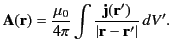(661)

Assuming that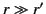, so that our observation point lies well outside the distribution, we can write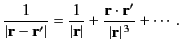(662)

Thus, the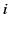th Cartesian component of the vector potential has the expansion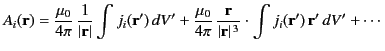(663)

Consider the integral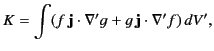(664)

where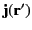is a divergence-free [see Equation (618)] localized current distribution, and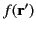and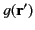are two well-behaved functions. Integrating the first term by parts, making use of the fact that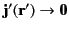as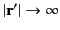(because the current distribution is localized), we obtain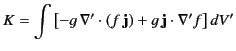(665)

Hence,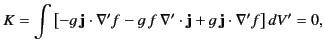(666)

because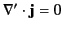. Thus, we have proved that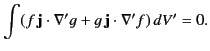(667)

Let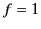and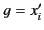(where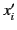is theth component of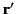). It immediately follows from Equation (668) that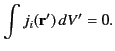(668)

Likewise, if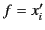and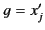then Equation (668) implies that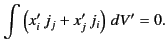(669)

According to Equations (664) and (669),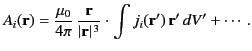(670)

Now,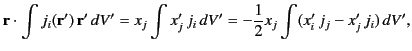(671)

where use has been made of Equation (670), as well as the Einstein summation convention. Thus,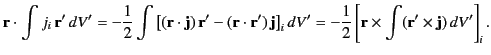(672)

Hence, we obtain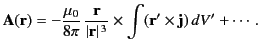(673)

It is conventional to define the magnetization, or magnetic moment density, as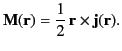(674)

The integral of this quantity is known as the magnetic moment: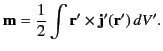(675)

It immediately follows from Equation (674) that the vector potential a long way from a localized current distribution takes the form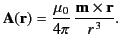(676)

The corresponding magnetic field is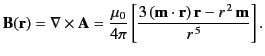(677)

Thus, we have demonstrated that the magnetic field far from any localized current distribution takes the form of a magnetic dipole field whose moment is given by the integral (676).

Consider a localized current distribution that consists of a closed planar loop carrying the current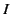. If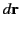is a line element of the loop then Equation (676) reduces to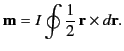(678)

However,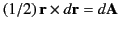, where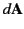is a triangular element of vector area defined by the two ends ofand the origin. Thus, the loop integral gives the total vector area,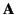, of the loop. It follows that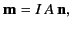(679)

where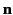is a unit normal to the loop in the sense determined by the right-hand circulation rule (with the current determining the sense of circulation). Of course, Equation (680) is identical to Equation (659).Next: Exercises Up: Magnetostatic Fields Previous: Circular Current Loop
Richard Fitzpatrick 2014-06-27